# Artificial Neural Networks with cWB¶Attention

Currently the ANN software is not maintained, most is outdated and are reported only as reference.

How to make and use Artificial Neural Networks (ANNs)

 Introduction: What are ANN: Artificial Neural Networks

From cWB output to ANN creation

 TF selection: extract the TF representation of the events from cWB 2G analysis; Matrix Conversion: convert the TF representation of each event in a NDIM \times NDIM matrix; Information collection: save all the useful information in a root-file;

ANN creation and usage

 ANN Creation: tools for the ANNs creation; ANN Use: tool for the ANN applications on different data sets; ANN Average: tool for the application on different data sets of more ANNs (averaging their outputs); ANN Tests: tools for deeper analysis;
All the files involved can be found in the cWB git repository in the library/tools/cwb/ann directory.

## Introduction¶

In the near future the new generation of interferometers will become operative with better sensitivities and we should be ready for a signal identification. The most important topic concerning the data-analysis is the discrimination between astrophysical and noise signals. Different strategies have been tested, one of which is the recognition of the signal traces in the time-frequency plane performed by ANNs.
Here is explained how to create and to use ANNs to recognize a generic shape in the time-frequency plane. The performances can vary a lot acconding to the characterization of the tested signals in the time-frequency plane. A defined signal is generally well identified by ANNs. This is for example the case of signals consistent with compact binary coalescences.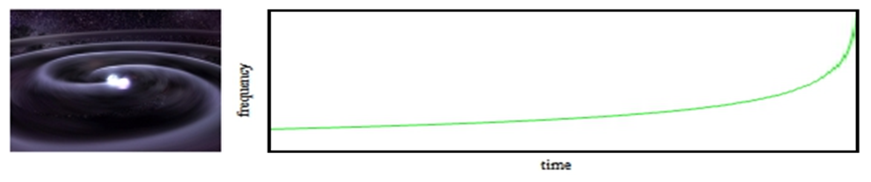*Compact binary coalescence: an example of interesting gravitationa wave source and its typical emission in the TF plane.*

Main idea: the cWB 2G pipeline analizes the data privided by the intereferometers, using a decomposition of the time-frequency plane with different resolutions. It reaches a complete representation of signals made up of time-frequency (TF) pixels belonging to different levels. A Plug-in was implemented to extract this information. Then an algorithm converts the selected pixels in a square-matrix with default dimensions 8x8. After having collected several examples of signal and background events (which represent respectively the class of TF-traces you want to recognize and the one you want to discard) is possible to create an ANN able to identify the target shape. Finally you can test the ANNs defined on different sets of events and verify how they work. In case of chirp-like signals this step also allows you to compare the ANN results to a fit strategy. Several other option had been tested, such as the simultaneous usage of more ANNs and the comparison between two different ones.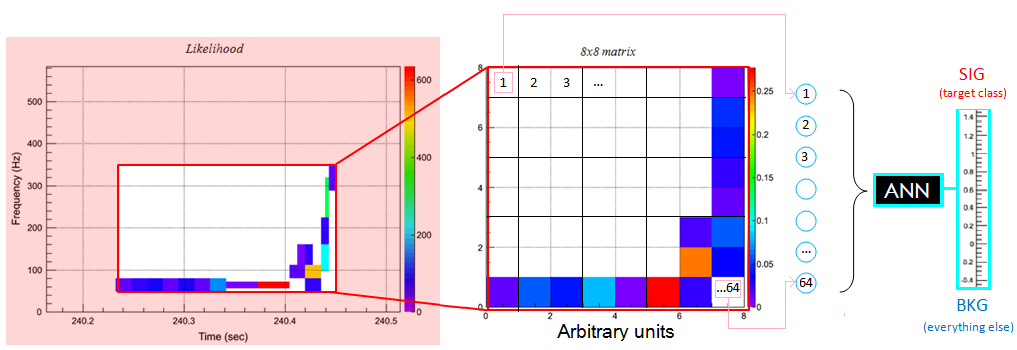## What are ANN¶

WHAT ARE ANNs?
The Artificial Neural Networks (ANNs) are algorithms which mimic the human brain activity using calculation units (neurons), connected together by synapses, represented by values (weights). In analogy with human brain, these structures learn the desired behavious from exaples: the ANN associates to each synapse a weight, which is set according to an optimization procedure based on a training set of examples (contained in the file otabined by the previous steps).
The ANNs are widely used for two main purposes: the association of different information and the interpretation of the inputs. Solving classification and regression problems belongs to the latter case. To reach these goals different algorithms are available, which differs in:
• neuron definitions: neurons are characterized by a threshold, which is generally treated as a surplus weight with default input equal to -1, and by an activation function which collectd all the neuron inputs to return an output. Different function can be used to define the neurons and the sigmoid functions are the most common.
• connection properties:
• feedback networks: the connections can have both the direction or anyway some neurons comunicate toward the inputs;
• feedforward networks: the connections have only one direction.

WHAT ARE MULTI-LAYER-PERCEPRTONs?

Multi Layer Perceptrons a particular feedforward ANNs where the neurons are organized in groups called layers. There are no connection between neurons belonging to the same layer. There exist three typs of layers:

• input layer: its task is to define the input quantities and pass them to the successive layer. The neurons belonging to this layer are said inactive because their transfer function is 1;
• hidden layers: each neurons of these layers calculates the weightened sum of its inputs and the correspondent output through the activation function. The inputs of such calculation units are the output quantities of the neurons bolonging to the previous layer. The user can use how many hidden layers he desires;
• output layer: the neurons belonging to this group act on their inputs as the ones which define the hidden layers. Their output values should be similar to the desired ones, according to the belonging class of events. This result can be reached with an appropriate training procedure.</li>
This kind of ANNs is the most commonly used for classification problems.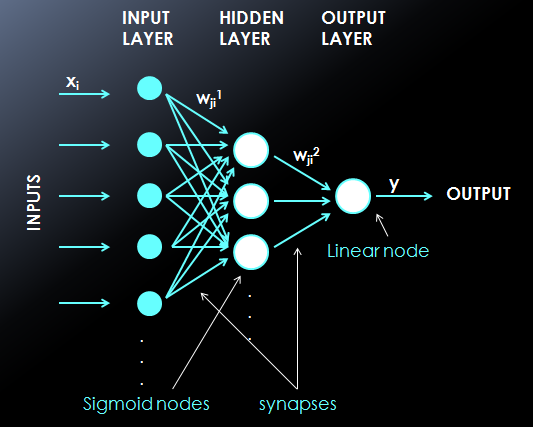RECOGNITION OF TF-TRACES

ANN architecture

• Input layer: it is constituted by neurons. The input quantities are the values of the normalized-likelihood calculated for each TF interval in the matrxi conversion step. Each elements of the resulted frame correspond to an ANN input neurons.
• Hidden layer: the number of these layers and the one of their neurons are free. The neurons are define by a sigmoidal activation function.
• Output layer: a single neuron, characterized by a linear activation function, is used to provide the final output value. The chosen choice is to train ANNs with desired output 1 for events belonging from the targer class, 0 otherwise.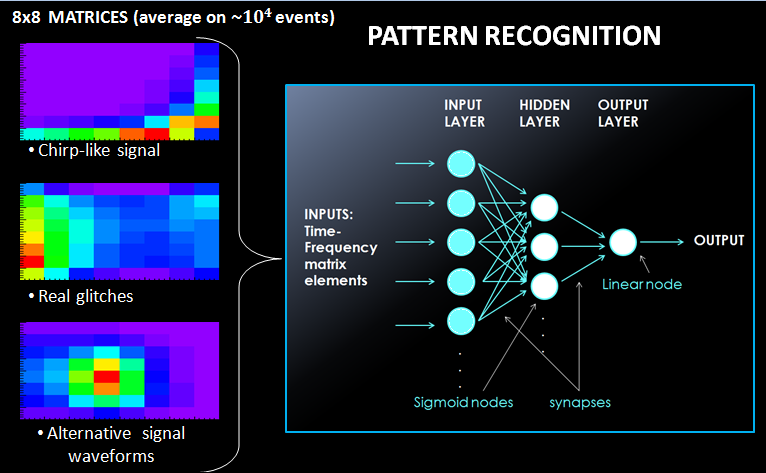Other information
The weight and threshold values are defined by the training procedure based on a learning techinque led by the provided examples. Their starting values are set randomly by an uniform distribution defined within the values -0.5 and 0.5.
The learning method applied for the parameter estiamtions is decided by the user among the six options provided by the ROOT-toolkit (see the TMultiPerceptron official ROOT page and a brief introduction on ANN learning methods). Another normalization is applied on the ANN input using a particular command in the ANN definition. In this way a function extract from the examples used during the trainin process a law to convert each single input in a new normalized value. Each of the linear relations is defined associating respectively -1 and 1 to the minimum and the maximum values, of the considered input, in the training set.

## TF selection¶

The plugin is a C++ function which is called by the pipeline different stages of the analysis and can be used to customize the analysis (see Plugins)

MAIN CHARACTERISTICS

extract information about the TF representation of the events. The cWB 2G analysis is based on the TF decomposition of the signals through the Wilson-Daubechies-Meyer (WDM) TF transformation in different TF resolutions (levels). The analyzed levels are chosen by the users in the user_parameters.C file. The final event recostruction in the TF plane is given by a collage of the most energetic pixels in the different levels. To male this representation complete and independent when a pixel is selected its contribute is removed by the other levels using appropriate tables.
Information saved:
a complete and independent representation of the event composed by selected pixels bolonging to different TF levels.
Plugin type:
CWB_PLUGIN_OLIKELIHOOD.
Extra information:
it can be used also to enable event monster plots and to introduce simulated noise and waveforms. To perform these addictional tasks the code uses different plugin types, acting in different moments of the cWB 2G analysis.

The standard Plugin is reported here:
• CWB_Plugin_NN.C
The CWB_Plugin_NN_Config.C and CWB_Plugin_BRST_Config.C files can be used to define the injected waveforms and their parameters. The former refers to LAL simulations, whereas the latter includes the Standard Simulation Set for burst signals.
USAGE
Available only for 2G analyses

To extract the necessary information from the 2G analysis you need to have in the “macro” directory of the simulation the following files:

• CWB_Plugin_NN.C strictly necessary for the information extraction;
• CWB_Plugin_NN_Config.C or CWB_Plugin_BRST_Config.C these files or similar ones are used to define the parameters of the waveforms you are interested in.
To use these macros the following lines must be declared in the user_parameters.C file:

plugin=TMacro("macro/CWB_Plugin_NN.C");
plugin.SetTitle("macro/CWB_Plugin_NN_C.so")
configPlugin=TMacro("macro/CWB_Plugin_NN_Config.C");


## Matrix Conversion¶

TURN THE TF-REPRESENTATION OF AN EVENT IN A FRAME

To use ANN for pattern recognition it is necessary to convert the TF representation of an event in a matrix whose elements constitute the ANN inputs. The algorithm implemented performs a conversion of the TF representation of the event in a square matrix.

 Legend number of ANN inputs: $$nINP$$ frame dimension in time: $$DIM_T$$ frame dimension in frequency: $$DIM_F$$ Useful relations $$nINP=DIM_T\times DIM_F$$ matrix dimension $$NDIM=nINP^{0.5}$$ $$NDIM=DIM_T=DIM_F$$
Default set
$\ nINP=36 \ NDIM=8$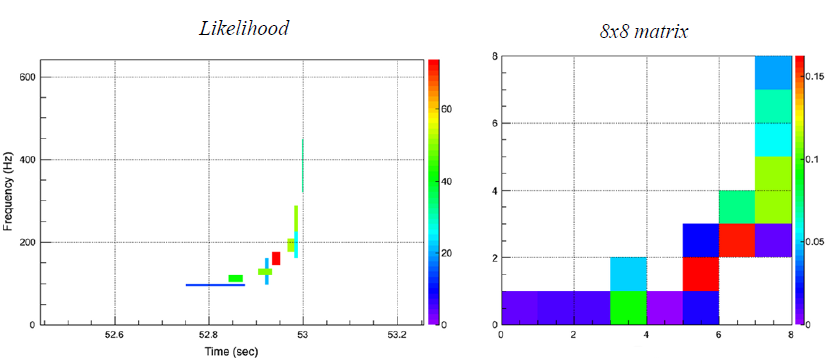Big-Dog event

For each event, the TF representation is composed of pixels selected by cWB 2G. They are well defined in time and frequency: each of them belongs from a particular decomposition level characterized by fixed resolutions ($$T$$Therefore different levels can be involved. Unfortunalety the time and frequency resolutions are connected in every level by the relation: $$T \times \delta f=0.5$$Each of these selected pixels is also characterized by a likelihood value different from zero. This is the key quantity which defines the ANN inputs. To use this information for the ANN training you can imagine the TF representation of an event as an image which will be treated in the following way.

How it works

ZOOM:
individuation of the time and frequency limits (respectively [$$T_i$$and [$$F_i$$defining $$\Delta T=T_f-T_i$$$$\Delta F=F_f-F_i$$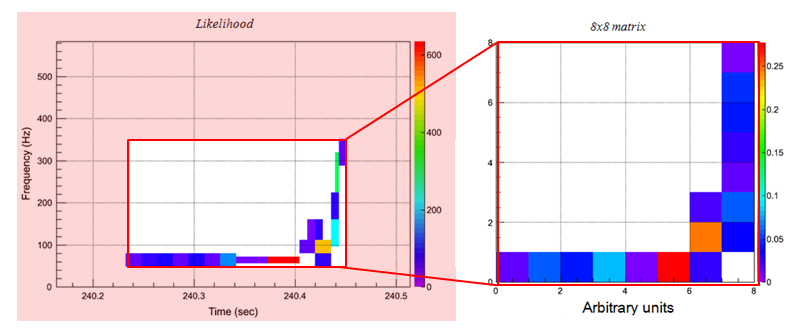PIXEL REMOVAL:

for the chirp recognition it is useful to remove some selected pixels if they satisfy determined conditions. Indeed if the analysis selects a noisy pixel after the chirping time it strongly modifies the resulted matrix and therefore comprimises the ANN performances. The process, by which the pixels are removed, is descrided in the following.

• The macro singularly considers the pixels according to the order of their central times starting from the last o>e. The pixel considered is removed if the cumulative likelihood is less than the 10\% of the one of the whole event. The cumulative likelihood is calculated summing the likelihood value of the considered pixel to the ones of the pixels just removed.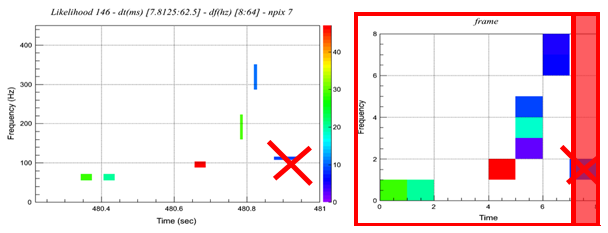FRAGMENTATION IN FUNDAMENTAL UNITS:

individuation of the smallest resolutions, in time $$T_{min}$$frequency $$\delta f_{min}$$analysis. The best resolution are determined by the decomposition levels $$L$$during the analysis: $$T=2^L\cdot r_s^{-1}$$$$r_s$$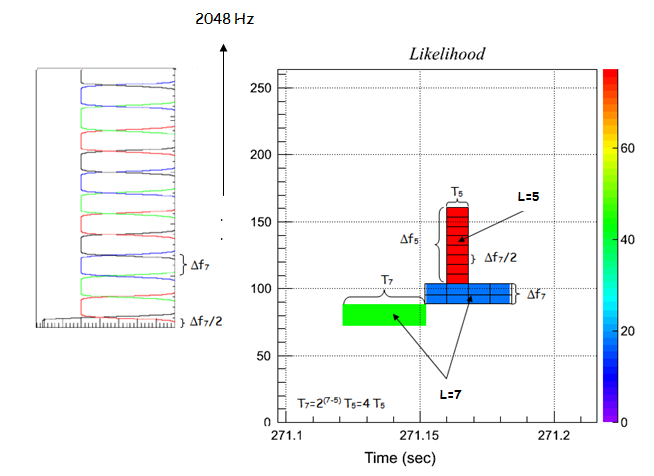MATRIX CREATION:

the image is converted in a matrix with the desired dimensions ($$DIM_T$$representation (the frequency one is treated at the same way), different processes can be adopted depending on the value of $$n_T=\Delta T\cdot (T_{min})^{-1}$$

• If $$n_T=l_T\cdot DIM_T$$
• else two procedures can be applied according to the value of $$d$$defined by the relation: $$(n_T+d)=l_T\cdot DIM_T$$
• if $$d<4$$(increasing the $$T_f$$(decreasing the $$T_i$$starting from the right one: $$T_i=T_i-int(d/2)\cdot T_{min}$$$$T_f=T_f+[d-int(d/2)]\cdot T_{min}$$$$T_f-T_i=(T_f-T_i)+d\cdot T_{min}[d-int(d/2)]\cdot T_{min}$$
• else the macro remove the necessary $$DIM_T-d$$(because
for our purpose of chirp beahviour recognition this is the less invasive choice). However the process consists also in adding the likelihood value of each removed pixel to the one associated to the first time-interval: $$T_i=T_i+(DIM_T-d)\cdot T_{min}$$$$T_f-T_i=(T_f-T_i)-(DIM_T-d)\cdot T_{min}[d-int(d/2)]\cdot T_{min}$$
• If $$n_{T(F)} < DIM_{T(F)}$$
Then the matrix and therefore the ANN inputs are defined in the following way: the fundamental units are divided in groups of $$l_T\times l_F$$likelihood values is calculated. This will be the ANN input value. Finally all the ($$DIM_T\times DIM_F$$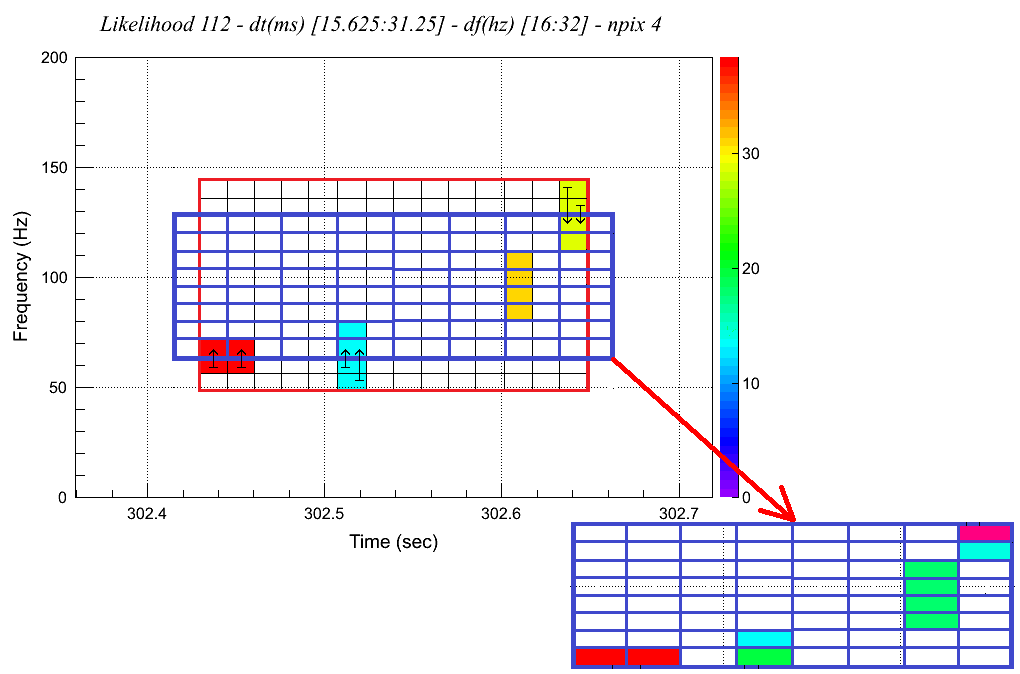Usage

The file used to convert the TF representation of an event to a frame is called
• ClusterToFrameNew01_dtmin_approx_cent.C

It requires as two strings as argument:

root -l -b 'ClusterToFrameNew01_dtmin_approx_cent.C+("in_path/in_file.root","out_path/out_file.root")'


The first has to be the name of the root file provided by the cWB 2G analysis (this means the name of a single job file or the name of the merge file), whereas the second has to be the desired name of the root output file.

If you want to join together different results you can use the
• MergeTrees.C

This macro has no argument, but you have to fill it with the right data (directory containing the files to merge, output directory and label of the output file), following the instructions you can find opening it. The tree name is just rightly written.

root -l -b MergeTrees.C


## Information collection¶

To train the ANNs other information must be added to the out-files obtained by the previous steps and the events of the two classes must be joined in a sigle file.

What is it

The instrument is implemented to train ANNs able to discriminate a particular kind of gravitational waves from the other events (above all from the ones with noisy origin). For this reason two different groups of events are needed, one associated to the target signals (SIG) and one representative of events with different nature, which will be called “background” (BKG). The main properties of the events of the two groups are saved in the two files obtained by the previous steps.
Now you have to use the macro
• TreeTest_CTFmod.C
to construct a file suitable for the creation of an ANN using the tools here explained. The main task of the code is to put together the two files collecting information useful for the ANN creation. For this reason the algorithm extracts and adds to the new root file the necessary information for the ANN definition. For each event the most important parameters introduced in the output files are:
• the $$nIMP$$
• the matrix dimension ($$NDIM=DIM_T=DIM_F$$thought to convert the TF representation of the events in a square matrix;
• the classification tag. It is a integer variable, called “type” (see here), used to define the target class. It represents the desired output and in the implemented case and therefore it assumes the value of “1” when the events belongs to the target group, “0” otherwise.<

Further secondary information are saved; here is the list: the names of the two input files, the duration of the last 20 selected pixels, the duration of the event, its central frequency, its central time, the applied option on the amplitudes (explained in the following), an index, the event number of the belonging class and the event weight.

Usage
The script
• TreeTest_CTFmod.C

requires some arguments:

        root -l -b 'TreeTest_CTFmod.C+("SIG_merge.root","BKG_merge.root","nnTREE/out_file.root",0/1,NDIM,nINP)'

- **SIG_merge.root**: the path and the name of the file which
contains the events belonging to the target class.
- **BKG_merge.root**: the path and the name of the file which
contains the events belonging to the background group.
- **nnTREE/out_file.root**: the path and the desired name of the
output file.
- **0/1**: it represents what was called applied "option on the
amplitudes", indeed it describes the amplitudes treatment.

-  Instead of *1* you can write any integer number, except *0*,
obtaining the same results: the normal consideration of the
selected pixel amplitudes. In this way the coordinate, that
determines the ANN inputs, can assume a continue scale of value.
-  However you can also adopt a different approach, choosing and
writing as argument the number *0*. In this way the number
associated to each matrix element (normalized-likelihood for fixed
time and frequency intervals) becomes a binary variable, whose
value is set to 0 if originally it was less than 0.001, to 1
otherwise.

- The integer **NDIM**: this value represents the desired matrix
dimension.

The choice of \$$DIM = 8 \$$therefore it has been deeply
investigated. Anyway remember that it must be chosen consistently
with the matrix dimension used to convert the TF representation of
the events.

- The integer **nIMP**: it represents the desired number of ANN
inputs. Having implemented a conversion in a square matrix its value
has to be \$$NDIM^2 \$$. Obviously also in this case, it must be
consistent with the choices defined in the previous steps.


NOTE: Some macros implemented for applications require this file to be in a directory whose name end with “nnTREE”, therefore you are invited to built such a directory and to save the output file there.

## ANN Creation¶

Necessary files: to create ANNs, with cwbANN_purity_BoS.C or cwbANN_purity_SoB.C it is necessary a file created following the previous steps. From this files a group for examples of both the classes (SIG and BKG) are selected to train the ANN. Half of the selected events (Train sample) are effectively used to train the network, while the other half are used for a preliminary test (Test sample).
Some examples of such files are in the directory /home/waveburst/ANN/Summary/example/GaussinNoise_nnTREE (details in Details).

Algorithm core: ROOT-toolkit TMultiLayerPerceptron for ANN creation.

 ANN creation-Equipment: the particular enviroment which is necessary; ANN creation-commands: how to use the files and their required arguments.

### ANN creation-Equipment¶

To create ANNs with the cwbANN_purity_BoS.C or cwbANN_purity_SoB.C files some directories are required in the location where the command is used:

• NN: this directory collects the output root files which contain the trained ANNs (one for each created ANN);

• thresFiles: this directory collects files which contain some images and some data about the correctly and the wrongly classified events of the set provided for the training. To perform the count different thresolds on the ANN output are applied and the correspondent information is saved (one for each created ANN);

• selectTREE: this directory collects the files associated to the events selected for the ANN training. They contains the main properties of the events used to train the ANN (one for each created ANN);

• mlpa_canvas: this directory collects images (one for each created ANN) which represent some characteristics of the selected events.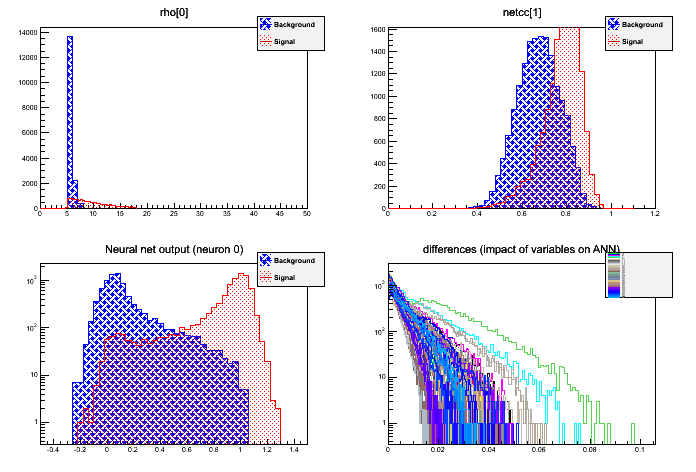Each saved picture is composed of 4 graphs which have in ordinate the number of the events. The histrograms on the top analyse the whole set of events, while the bottom ones refers to exaples of the Test sample. Summarizing:

• top-left (whole selected set):
ordinate = event cout, abscissa = cc (Network Correlation coefficient);
• top-right (whole selected set):
ordinate = event cout, abscissa = rho (effective correlated SNR);
• bottom-left (Test sample):
ordinate = event cout, abscissa = ANN output (red = SIG, blue =BKG);
• bottom-right (Test sample):
ordinate = event cout, abscissa = difference in ANN output, varying each input (1 input = 1 color)

• PropertyGraphs: this directory collects images (one for each created ANN) which represent the mean values of each frame elements of the set of events selected for the ANN training. | The first row concerns the whole set of selected events, the second the SIG ones and the third the BKG ones. The first column represents the RMS of each pixels of the matrix, the second the average and the third the variance.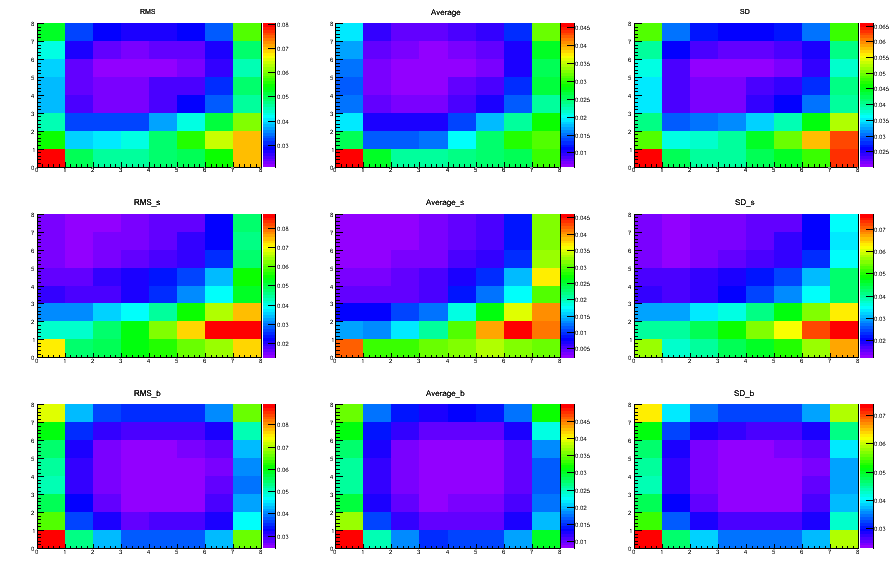• TRAIN_GRAPHS: this directory collects images (one for each created ANN) which contain 6 graphs (see the “LEGEND” table).• top-left (Train sample):
ordinate = True_Alarms/Total_SIG_events, abscissa = False_Alarms/Total_BKG_events;
• center-left (Train sample):
ordinate = True_Alarms/Total_SIG_events, abscissa = threshold on ANN output;
• bottom-left (Train sample):
ordinate = False_Alarms/Total_BKG_events, abscissa = threshold on ANN output;
• center-right (Test sample):
ordinate = True_Alarms/Total_SIG_events, abscissa = False_Alarms/Total_BKG_events;
• top-right (Test sample):
ordinate = True_Alarms/Total_SIG_events, abscissa = threshold on ANN output;>
• bottom-right (Test sample):ordinate = False_Alarms/Total_BKG_events, abscissa = threshold on ANN output;

• CC_OUT: this directory collects images (one for each created ANN) composed of 4 graphs. All the four pictures refer to the events of the Test sample. Three of them are scatter plots where the red-dots represent the <font color=”red”>SIG</font> events and the blue-points the <font color=”blue”>BKG</font> ones. The meaning of each graph is briefly explained in the following (see the “LEGEND” table):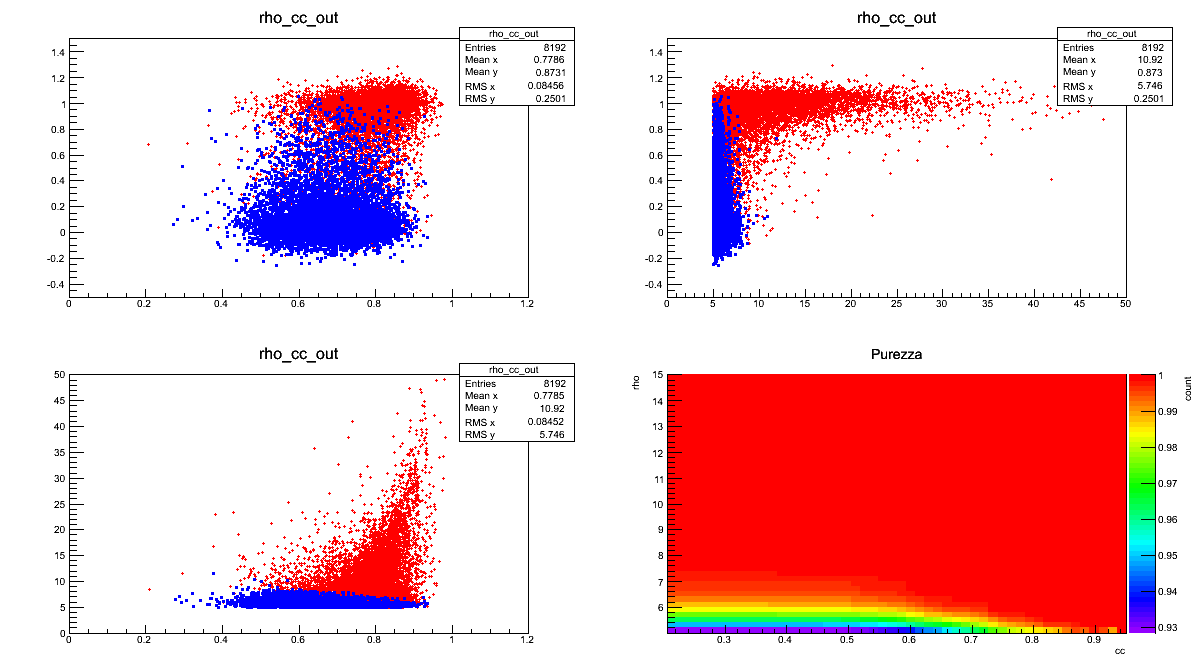• top-left:
ordinate = ANN output, abscissa = cc (Network Correlation Coefficient);
• top-right:
ordinate = ANN output, abscissa = rho (Effective Correlated SNR);
• bottom-left:
ordinate = rho (Effective Correlated SNR), abscissa = cc (Network Correlation Coefficient);
• bottom-right:
ordinate = statistical purity (True_Alarms/[True_Alarms + False_Alarms]).

• ErrorGraphs:
this directory collects images (one for each created ANN) where are represented the error-values (ordinate) in function of the epochs number.
This kind of pictures are useful to choose the number of epochs which better satisfies the compromise between gain and time. Be careful: an exagerate number of epochs can provoke a lost of generality and the algorithm can be able to recognize only the examples analysed during the training procedure. |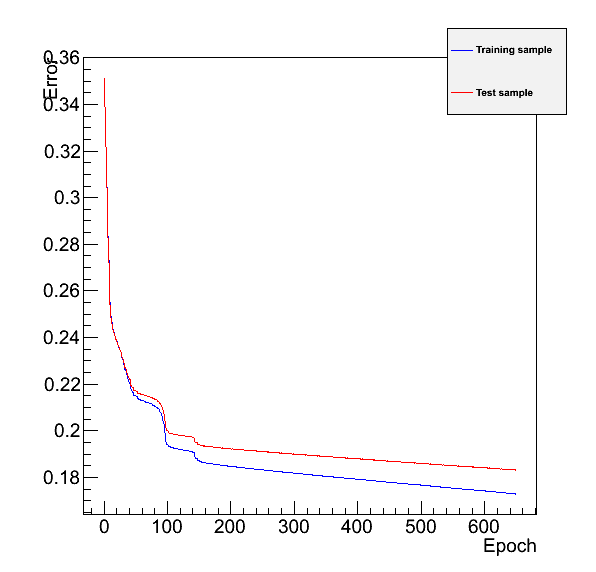|
The red-line refers to the Train sample, the blu-one to the Test sample. | |

LEGEND

Taget-Signal presence

Taget-Signal absence

Hypotesis of SIG presence

True Alarm

False Alarm

Hypotesis of SIG absence

False Dismissal

True Dismissal

WARNING:to be reliable the number of SIG-events and of BKG-events used to train the ANN must be equal. For the tests previously performed this configuration gives the best results.

The two files

• cwbANN_purity_BoS.C

or

• cwbANN_purity_SoB.C

which differ only in the superposition of the dots in all the scatter plots, can be found in the directory /home/waveburst/ANN/Summary/NNcreation, together with all the necessary directories. Here there are two addictional directories: the log and condor. These last are useful only if the ANN creations happen as jobs on clusters.

### ANN creation-commands¶

Use the
• cwbANN_purity_BoS.C

file if you want to create an ANN emphatizing the BKG events in the output scatter plots (easier view of the False Alarms); | Use the

• cwbANN_purity_SoB.C

file if you want to create an ANN emphatizing the SIG events in the output scatter plots (easier view of the False Dismissals). | | The general command is:

root -l -b 'cwbANN_purity_BoS.C+(nTrainS, nTrainB, "architecture", "in_file.root", initial_SIG,
initial_BKG, max_rho, max_cc, learning method, epochs)'

NOTE:: Both the files require the same 11-arguments. The last four arguments are not mandatory (they are defined by default), but be careful, especially with the last two ,because they are strictly linked to other important parameters.
ARGUMENT DESCRIPTION
• nTrainS: number of the examples belonging to the SIG class that will be used to train the ANN (whole set).

• nTrainB: number of the examples belonging to the BKG class that will be used to train the ANN (whole set).

• architecture: a string which defines the desired hidden layers, which are separated by colons, by fixing the number of their neurons. In this way the Multi-Layer-Perceptron architecture is determined, indeed the output and the input layers are fixed by the code and by the previous steps. Defined architecture:

• input layer with $$NDIM\times NDIM$$
• output layer with 1 output linearneuron.
• hidden layers with 3 hidden layers with respetively (from inputs to output): 16, 32 and 16 neurons.</li>
• in_file.root: this argument must define input file which contains the events that you are going to use to train the ANN. This file must be built following the previous steps.

• initial_SIG: this number establishes the first SIG-event selected to compose the Train and the Test samples. All the events in the input-file are tagged by an ordered increasing index, used to identify them. The other nTrainS-1 examples are selected from this starting point, following their order inside the input-file.

• initial_BKG: this number establishes the first BKG-event selected to compose the Train and the Test samples.The other nTrainB-1 examples are selected from this starting point, following their order inside the input-file. Having saved in a single input-file all the events, starting from the SIG ones, the index of this first BKG event must be greater than the total number of the SIG signals.

• max_rho: this argument defines the maximum rho (Effective Correlated SNR) represented in the purity-graph.

• max_cc: this argument defines the maximum cc (Network Correlation Coefficient) represented in the purity-graph.

• learning method: this parameter defines the learning method used to train the ANN. It is therefore an index which can take integer values in the interval [1,6]:

1. Stochastic minimization
2. Steepest descent with fixed step size (batch learning)
3. Steepest descent algorithm
4. Conjugate gradients with the Polak-Ribiere updating formula
5. Conjugate gradients with the Fletcher-Reeves updating formula
6. Broyden, Fletcher, Goldfard, Shanno (BFGS) method

• epochs: this argument determines the epoch number. This parameter fixes how many times the Train Set is considered before defining the final ANN structure, i.e. before fixing the weight and the threshold values.

You can find both the files in the /home/waveburst/ANN/Summary/NNcreation directory.

root -l -b 'cwbANN_purity_BoS.C+(16384,16384,"16:32:16","nnTREE/nnTree_CTF01_dtmin_app_cent_recSIG25_BKG.root",0,1200000,15,0.9,5,650)'
or

root -l -b 'cwbANN_purity_SoB.C+(16384,16384,"16:32:16","nnTREE/nnTree_CTF01_dtmin_app_cent_recSIG25_BKG.root",0,1200000,15,0.9,5,650)'

WARNING: the files used on the example are obtained by the last version of all the process, BUT the data were not correctly analysed in the cWB process. Thus don’t take care too much about the results.

## ANN Use¶

After having created an ANN you can test it on a sample of events belonging to the test-file, which can be the input-file (used to train the ANN) or to another one. To perform this test you can use the
• Test0_dANN_var.C
Through this file you can study the ANN performances on any set of events, analysing the ANN behaviour on large statistics. To achieve this knowledge different graphs are created.
NOTE: the test-file must have the same characteristics of the input-file, i.e. it must be created following the previous steps. For this reason it’s necessary to know the class (SIG or BKG) of each event.

Test on ANN

### File for Test an ANN-Explanation¶

• Test0_dANN_var.C
file is to analyse:
• the ANN performances
• the impact of the ANN output on the event evaluation
One of the main reasons of the ANN introduction is to improve the recognition of a particular kind of events against the other, lowering the noise impact at least on the considered class of signals. Using the - Test0_dANN_var.C
you can save and visualize the ANN output role in relation with the two most important parameters of the cWB post-production analysis: *cc* (Network Correlation Coefficient) and *rho* (Effective Correlated SNR).
MAIN PICTURES
Each test produces the following pictures. Every of them contains 4 graphs.
The first 4 pictures cointain 4 different graphs, each of them are characterized by a different threshold value on the cc parameter: $$cc_{th}=$$Only events with $$cc>cc_{th}$$survive the cuts and are taken into account in the graphs.

N_ANN_S_NN_*_FILE_*_dANN0*_uf*.png (only SIG events)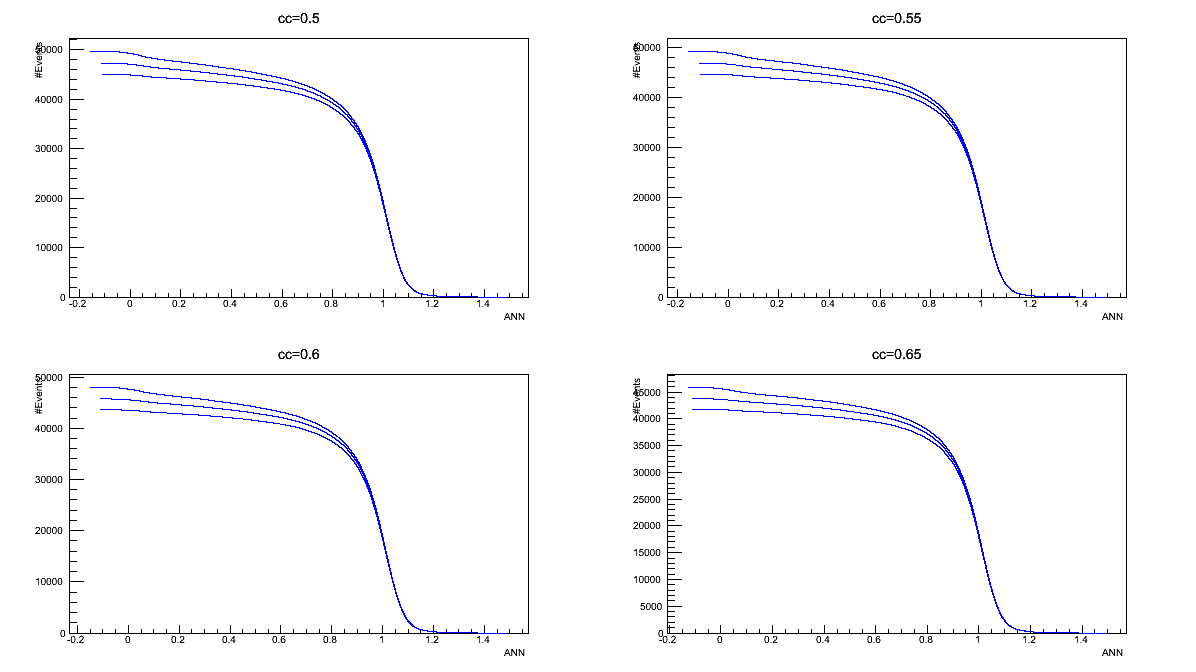• three different curves concern different thresholds on rho parameter: cc parameter: $$rho_{th}=$$survive the cuts and are taken into account in the graphs;

• ordinates = count of events survived to all the cuts (on cc, rho and ANN output);
abscissas = threshold values of ANN output ($$ANN output_{th}$$Only events with $$ANN output_{th}$$graphs;
N_ANN_B_NN_*_FILE_*_dANN0*_uf*.png (only BKG events)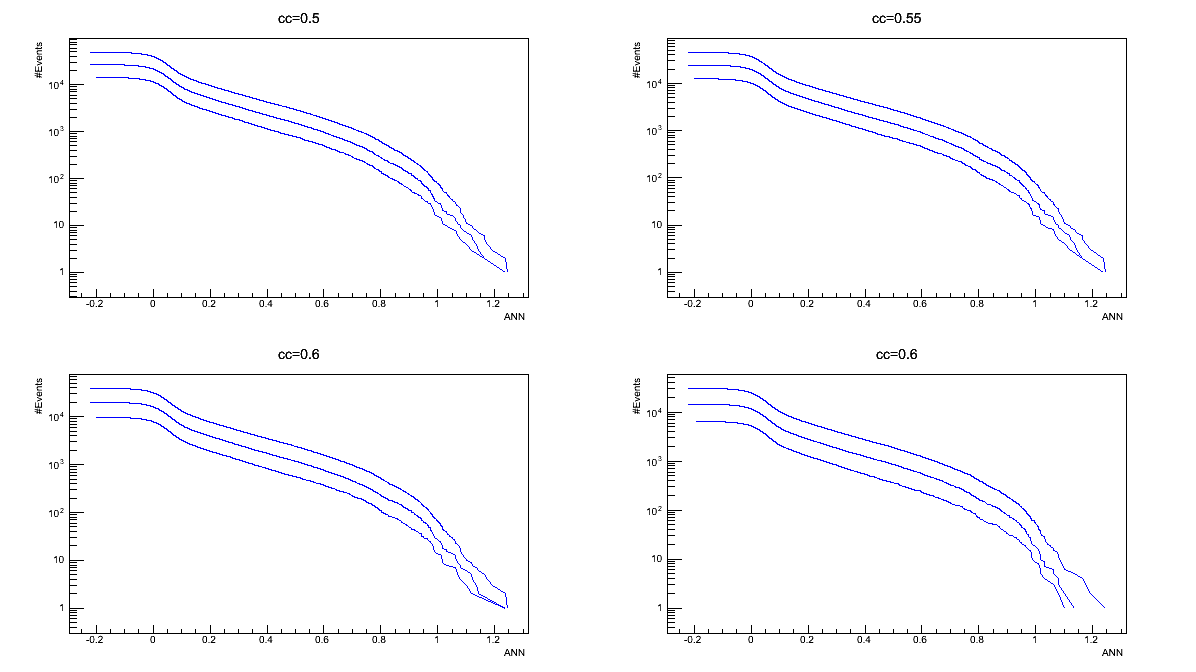• three different curves concern different thresholds on rho parameter: cc parameter: $$rho_{th}=$$survive the cuts and are taken into account in the graphs;

• ordinates = count of events survived to all the cuts (on cc, rho and ANN output;
abscissas = threshold values of ANN output ($$ANN output_{th}$$Only events with $$ANN output_{th}$$graphs;
logN_rho_S_dANN*_NN_*_FILE_*_dANN0*_uf*.png (only SIG events)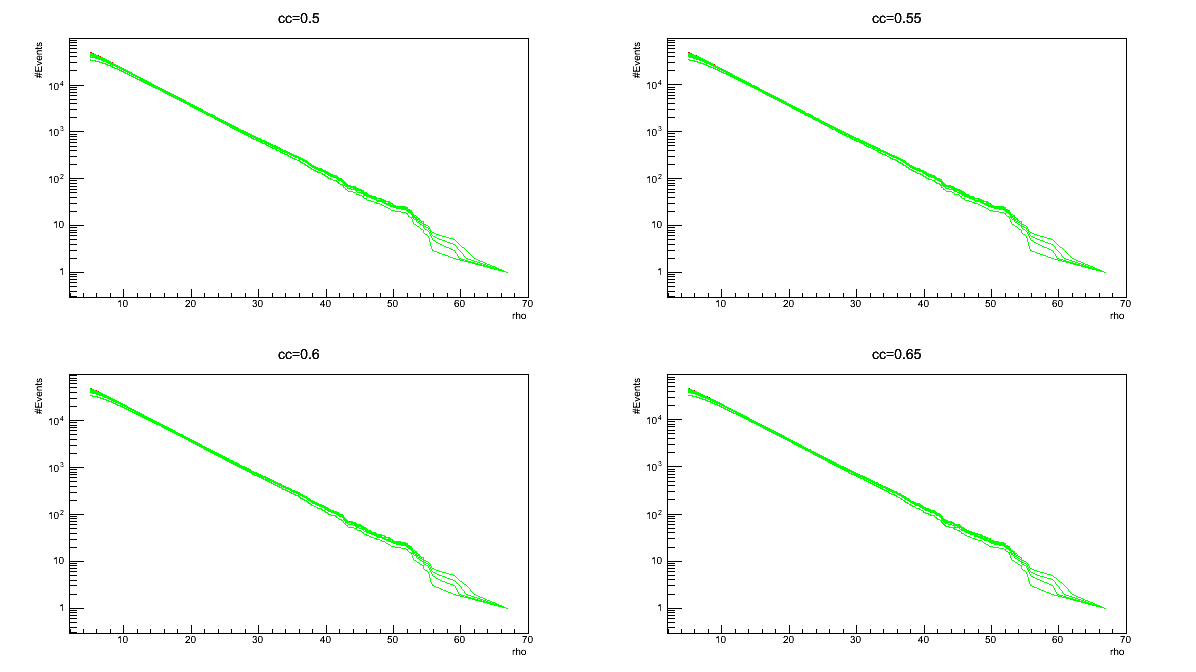• each graph contains ten different curves concerning different thresholds on ANN \ output parameter:

• to the red curve corresponds no cut on ANN \ output
• to the green curves correspond threshold values in the interval [0.2-1] with steps of 0.1;

Only events with $$ANN\ output>ANN\ output_{th}$$in the graphs;

• ordinates = count of events survived to all the cuts (on cc, rho and ANN output);
abscissas = threshold values of rho ($$rho_{th}$$Only events with $$rho>rho_{th}$$
logN_rho_B_dANN*_NN_*_FILE_*_dANN0*_uf*.png (only BKG events)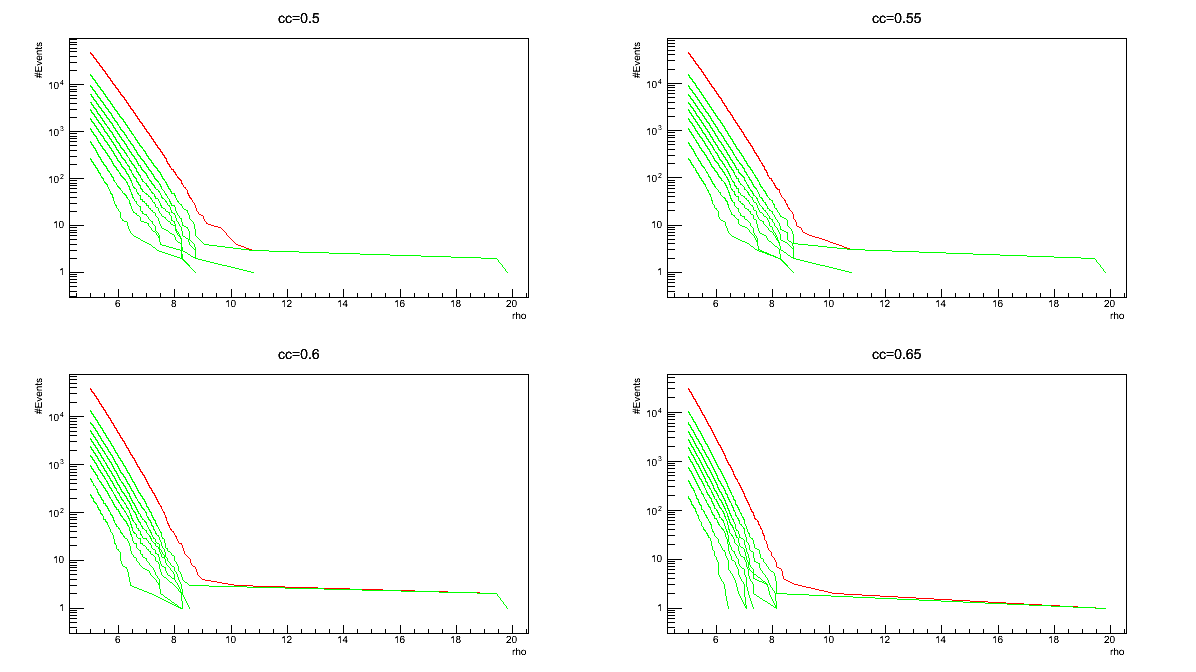• each graph contains ten different curves concerning different thresholds on ANN \ output parameter:

• to the red curve corresponds no cut on ANN \ output
• to the green curves correspond threshold values in the interval [0.2-1] with steps of 0.1;

Only events with $$ANN\output>ANN\ output_{th}$$in the graphs;

• ordinates = count of events survived to all the cuts (on cc, rho and ANN output);
abscissas = threshold values of rho ($$rho_{th}$$Only events with $$rho>rho_{th}$$
CC_RHO_ANN_Plots_NN_*_FILE_*_dANN0*_uf*.png (all events). The following first three graphs are scatter plots where the blue points refer to the BKG events and the red dots to >SIG the ones.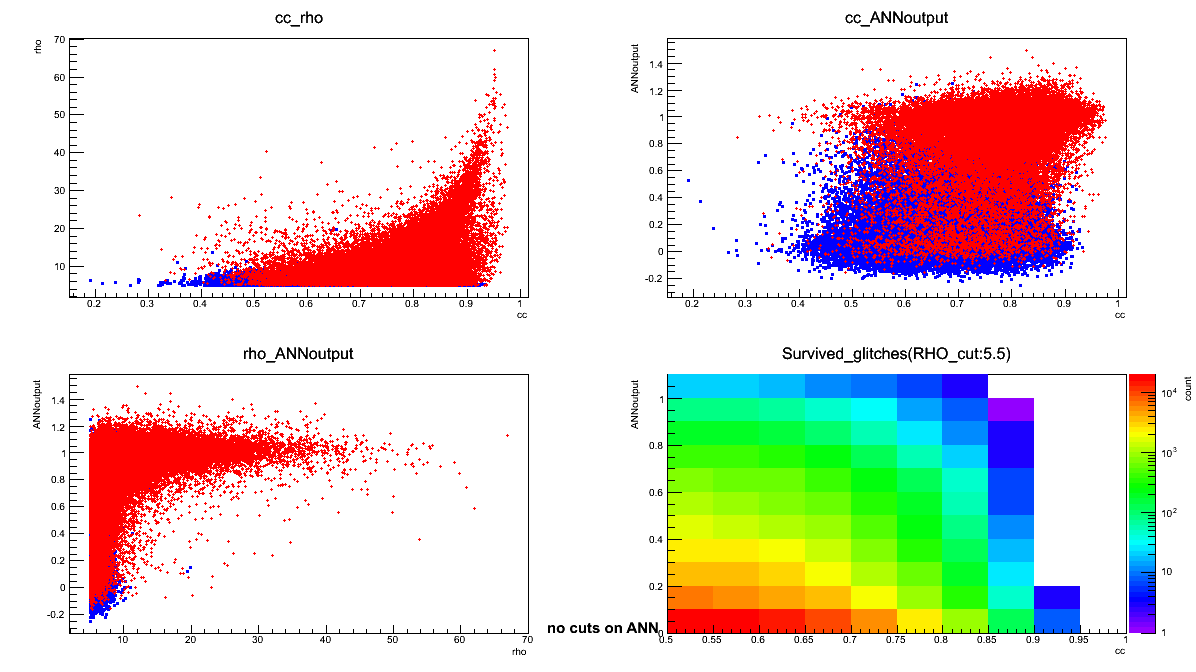• top-left:
ordinate = rho (Effective Correlated SNR), abscissa = cc (Network Correlation Coefficient);
• top-right:
ordinate = ANN output, abscissa = cc (Network Correlation Coefficient);
• bottom-left:
ordinate = ANN output, abscissa = rho (Effective Correlated SNR);
• bottom-right: color = count of survived BKG events,
ordinate = threshold value of ANN output ($$ANN\ output_{th}$$Network Correlation Coefficient).
Only events with $$rho>5.5$$$$ANN$$survive the cuts and are taken into account in the graphs.

The picture names contain the (*) symbol to sign the presence of string which depends on the peculiar characteristcs of the test. In all the cases the

* replaces the name of the root-file which contains the ANN; * replaces the name of the root-file which contains the events for the test; * replaces the ANN output interval used for the creation of the 3. and 4. pictures; * replaces an index (uf) value which is: 0 if the test-file is not the input-file; any other number otherwise.

### File for Test an ANN-Use¶

To use the - Test0_dANN_var.C file and test the ANN you need to know:

ANN-Equipment

In the location where the file and test the ANN you need to know: file is used, the following directories must exist:

• outfile: this directory collects the output files (one for each test) which contain the data;
• ANNthres: this directory collects the pictures 1 and 2 at this section.
• logN_rho: this directory collects the pictures 3 and 4 at this section.
• CC_RHO_ANN_Plots: this directory collects the picture 5 at this section.
NOTE:
The ANN file must come from a directory whose name ends with NN.
The test-file must come from a directory which ends with nnTREE.

ANN-commands

The generic command is:

root -l -b 'Test0_dANN_var_norm.C+("path/..NN/NN_FILE.root","path/..nnTREE/TEST_FILE,
# SIG-events for test, # BKG-events to test, start-SIG, start-BKG, uf)'

WARNING: the files used on the example are not obtained by the last version of the files used in the previous steps.
Only the first two arguments are mandatory; the other are set to 0 by default (see meaning in the following).
You can try exactly this command from the directory /home/waveburst/ANN/Summary/example/ANNTest.

ARGUMENT DESCRIPTION
path/..NN/NN_FILE.root: this argument defines the file which contains the ANN which you are going to test. path/..nnTREE/TEST_FILE: this argument defines the test-file which contains the events that you are going to analyse. This file must be built following the previous steps. # SIG/BKG-events for test (TS/BG): this number defines how many examples belonging to the SIG/BKG class you are going to analyse to test the ANN performances. The default value is set to 0; when TS=0 and TB=0 the number of the considered events is the minimum between the total ones of the SIG and the BKG classes. start-SIG/BKG (s/b): this number establishes the first SIG/BKG-event selected to test ANN. All the events in the test-file are tagged by an increasing index, used to identify them. The other TS-1/TB-1 examples are selected from this starting point, following their order in the test-file. The default value is set to 0; when s=0/b=0 the first considered SIG/BKG-event is the first of the list. uf: this number represents an index value which must be: 0 if the test-file is not the input-file; any other number otherwise.
root -l -b 'Test0_dANN_var.C+("NN/8x8Sig_Bkg_mlpNetwork_nTS16384_nTB16384_structure16_32_16_lm5_epochs650_s0_b1200000_ROC.root",
"nnTREE/nnTree_NN_CFT01mod_GaussianNoise_SIM_run3_BKG.root",10,10,0,0,0)'


You can find the - Test0_dANN_var.C file and the necessary directories here: /home/waveburst/ANN/Summary/TEST/TestANN.

## ANN Average¶

To improve the SIG and BKG discrimination can be use the - Average.C The main task of this script is to use several ANNs to final judge each event. To achieve this aim the outputs are averaged and the result is use to classify the event. |

### File for averaging ANN outputs-Explanation¶

The main task of the Average.C file is to calculate for each event the average of some ANN outputs. For every example the file also calculates the standard deviation on the ANN ouputs. The Average.C file allows an average on 8 or less ANN outputs.
To use this file you must know the class of each event.
Six pictures are created for every test sample. The first 4 are similar to the 1.-4. of this section ones produced to test a single ANN. The difference is the use of the average on the ANN outputs, instead of the output of a single ANN.

MAIN PICTURES
All the produced pictured are saved both in png and in root extensions. The first 4 1.-4. pictures cointain 4 different graphs, each of them are characterized by a different threshold value on the cc parameter: $$cc_{th}=$$$$cc>cc_{th}$$In each of the last two pictures are represented two scatter plots of the tested events. The SIG events are represented by red points, while the BKG ones are associated to the blue dots. The graphs on the top show the red dots on the blue ones, viceversa in the bottom plots.

N_ANN_S_*.png (only SIG events)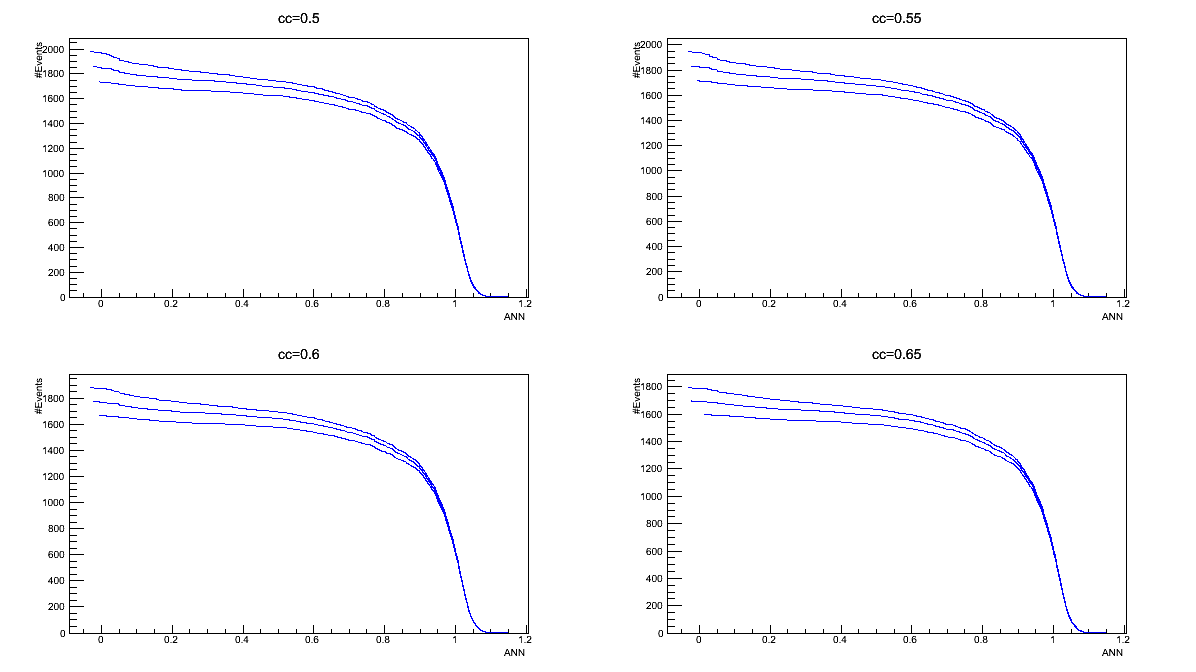• three different curves concern different thresholds on rho and cc parameter: $$rho_{th}=$$ survive the cuts and are taken into account in the graphs;

• ordinates = count of events survived to all the cuts (on cc, rho and average on ANN outputs);
abscissas = threshold values of average on ANN outputs ($$ANNaverage_{th}$$Only events with $$ANN average>ANNaverage_{th}$$account in the graphs;
N_ANN_B_*.png (only BKG events)• three different curves concern different thresholds on rho and cc parameter: $$rho_{th}=$$ survive the cuts and are taken into account in the graphs;

• ordinates = count of events survived to all the cuts (on cc, rho and average on ANN outputs);
abscissas = threshold values of average on ANN outputs (\ ANNaverage_{th} \)Only events with $$ANN average>ANNaverage_{th}$$account in the graphs;
logN_rho_S_dANN_*.png (only SIG events)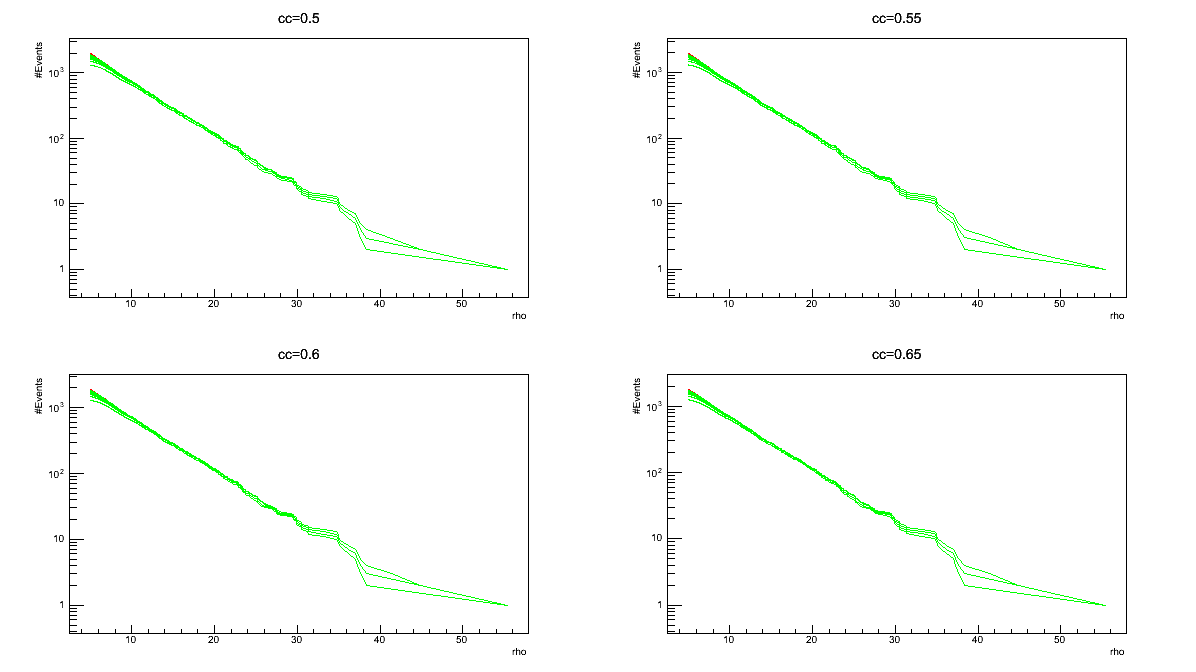• each graph contains ten different curves concerning different thresholds on ANN average parameter:

• to the red curve corresponds no cut on ANN average;
• to the green curves correspond threshold values in the interval [0.2-1] with steps of 0.1;

Only events with $$ANNaverage>ANNaverage_{th}$$

• ordinates = count of events survived to all the cuts (on cc, rho and average on ANN outputs);
abscissas = threshold values of rho ($$rho_{th}$$Only events with $$rho>rho_{th}$$
logN_rho_B_dANN_*.png (only BKG events)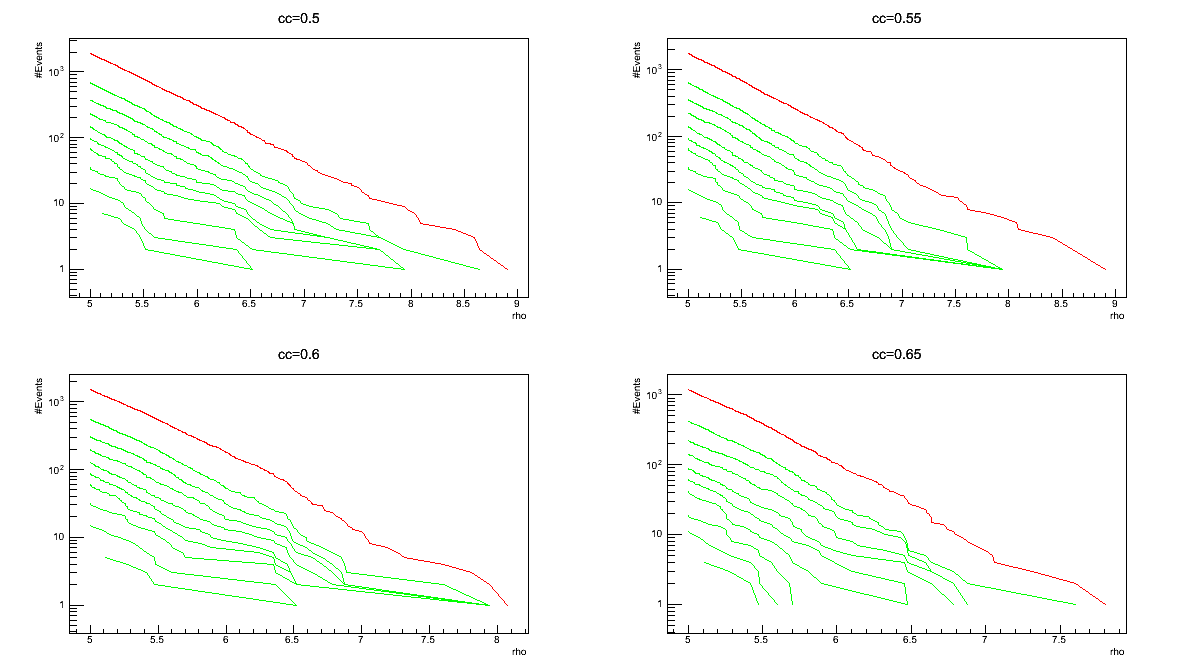• each graph contains ten different curves concerning different thresholds on ANN average parameter:

• to the red curve corresponds no cut on ANN average;
• to the green curves correspond threshold values in the interval [0.2-1] with steps of 0.1;

Only events with $$ANNaverage>ANNaverage_{th}$$

• ordinates = count of events survived to all the cuts (on cc, rho and average on ANN outputs);
abscissas = threshold values of
rho ($$rho_{th}$$Only events with $$rho>rho_{th}$$
Mc_Plots_*.png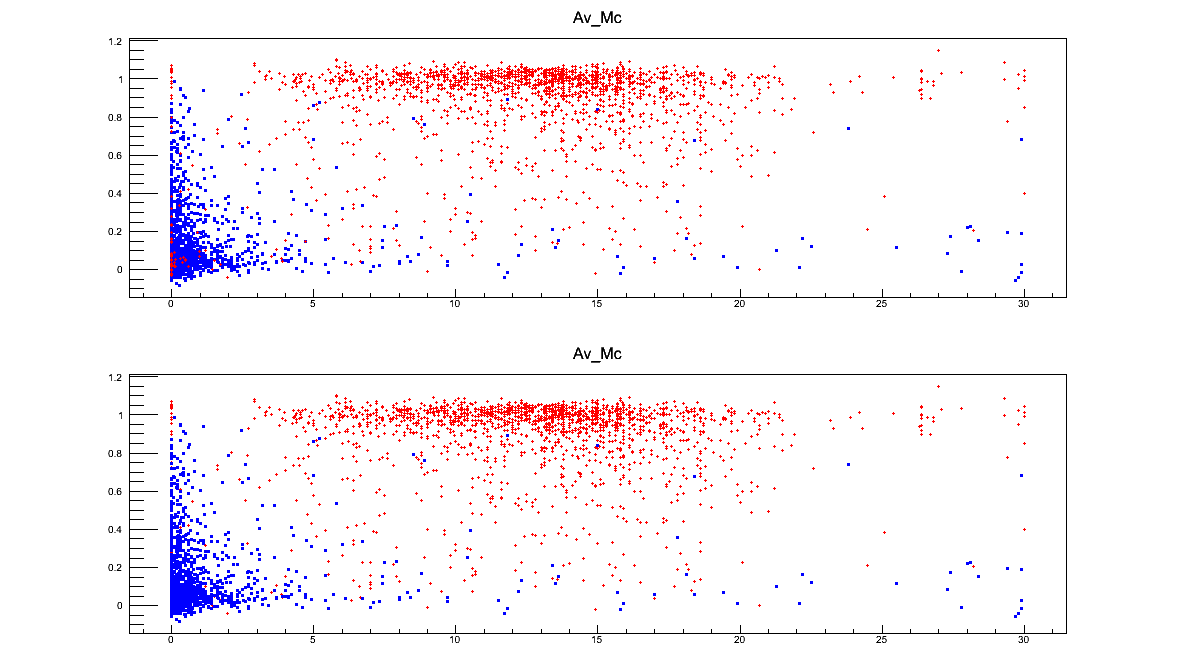• ordinates = average on ANN outputs;
abscissas = Mchirp
• Mchirp is an estimate of the chirp-mass, obtained through a fitting technique. Obviously this parameter and this comparison is useful only if you are interested in recognizing chirp-like events. The value of this parameter is always calculated for each event by the standard cWB analysis;

out_Plots_*

image135|

• ordinates = average on ANN outputs abscissa = cc

The picture names contain the (*) symbol to sign the presence of a string which depends on the decided output name of the test. It is defined as argument of the

• Average.C

## ANN Tests¶

Different other files are available to perform deeper tests. Here you find the list.
Files for deeper analysis
• the
• Test_rho_cc_out1_out2.C file in the directory *Comparison*: it can be used to compare the preformances of two different ANNs;
• Test0_dANN_var_norm.C and
• Test0_dANN_var_norm_neg.C files in the directory *Diff_NORM*: the elements of each frame are differently normalized according to their maximum and minimum values. The first file normalizes the inputs in a range [0,1] the second in the range [-1,1]
• Fisher_1.C file in the directory *Fisher*: this file create an algorithm which finds the Fisher distriminant between the BKG and the SIG events in the ANN output-cc plane. Then it tests this tool on another set of data;
• Test0_Mchirp.C file in the directory *Mchirp*: this file is the analogous of the Test0_dANN_var.C file but the analysis concern the Mchirp parameter instead of the ANN output.

NOTE: Inside each sub-directory there is the necessary equipment.
If you have any question about them, write to serena.vinciguerra89@gmail.com

You can find all these files in sub-directories of /home/waveburst/ANN/Summary/TEST.